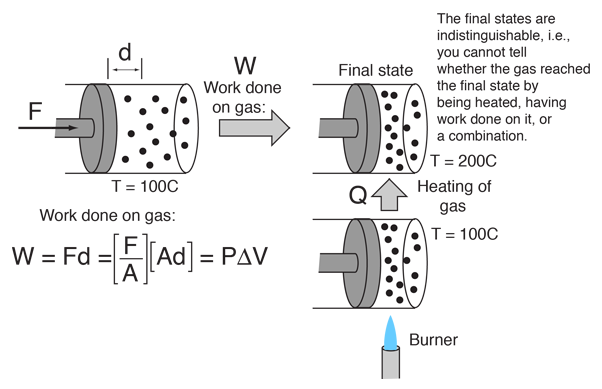# Heat

Heat may be defined as energy in transit from a high temperature object to a lower temperature object. An object does not possess "heat"; the appropriate term for the microscopic energy in an object is internal energy. The internal energy may be increased by transferring energy to the object from a higher temperature (hotter) object - this is properly called heating.Mechanical equivalent of heat
Index

Heat engine concepts

 HyperPhysics***** Thermodynamics R Nave
Go Back

# Heat and Work ExampleThis example of the interchangeability of heat and work as agents for adding energy to a system can help to dispel some misconceptions about heat. I found the idea in a little article by Mark Zemansky entitled "The Use and Misuse of the Word 'Heat' in Physics Teaching". One key idea from this example is that if you are presented with a high temperature gas, you cannot tell whether it reached that high temperature by being heated, or by having work done on it, or a combination of the two.

To describe the energy that a high temperature object has, it is not a correct use of the word heat to say that the object "possesses heat" - it is better to say that it possesses internal energy as a result of its molecular motion. The word heat is better reserved to describe the process of transfer of energy from a high temperature object to a lower temperature one. Surely you can take an object at low internal energy and raise it to higher internal energy by heating it. But you can also increase its internal energy by doing work on it, and since the internal energy of a high temperature object resides in random motion of the molecules, you can't tell which mechanism was used to give it that energy.

In warning teachers and students alike about the pitfalls of misusing the word "heat", Mark Zemansky advises reflecting on the jingle:

 "Teaching thermal physics Is as easy as a song: You think you make it simpler When you make it slightly wrong." Zemansky's plea Don't refer to the "heat in a body", or say "this object has twice as much heat as that body". He also objects to the use of the vague term "thermal energy" and to the use of the word "heat" as a verb, because they feed the misconceptions, but it is hard to avoid those terms. He would counsel the introduction and use of the concept of internal energy as quickly as possible.

Zemansky points to the First Law of Thermodynamics as a clarifying relationship. The First Law identifies both heat and work as methods of energy transfer which can bring about a change in the internal energy of a system. After that, neither the words work or heat have any usefulness in describing the final state of the sytem - we can speak only of the internal energy of the system.

 Mechanical equivalent of heat First law of thermodynamics Calculation
Index

Internal energy concepts

Reference
Zemansky

 HyperPhysics***** Thermodynamics R Nave
Go Back

# Mechanical Equivalent of Heat

Heat flow and work are both ways of transferring energy. As illustrated in the heat and work example, the temperature of a gas can be raised either by heating it, by doing work on it, or a combination of the two.

In a classic experiment in 1843, James Joule showed the energy equivalence of heating and doing work by using the change in potential energy of falling masses to stir an insulated container of water with paddles. Careful measurements showed the increase in the temperature of the water to be proportional to the mechanical energy used to stir the water. At that time calories were the accepted unit of heat and joules became the accepted unit of mechanical energy. The British Thermal Unit was also introduced. Their relationship to joules isThese conversions are the International Steam Table (IT) values, and variations up to 0.5% will be found since they were originally based on energy for temperature changes in water, and that varies a small amount based on what temperature of water is used to establish it. The heat capacity of water does change slightly with temperature.

 First law of thermodynamics
Index

Internal energy concepts

 HyperPhysics***** Thermodynamics R Nave
Go Back

# Constant Pressure WorkFrom the definition of work W=Fd for a constant force F acting along a distance d:For a constant pressure process only, the work is:For pressure P = Pa = x 10^ Pa = atmos
= mmHg = lb/in2,

a movement of d=m within a piston of radius r = m

gives a change in volume ΔV= m3 = cm3 =liters.

For the constant pressure case, this corresponds to a work
W = J = x10^ J.
 Heat and work example
Index

Heat engine concepts

 HyperPhysics***** Thermodynamics R Nave
Go Back